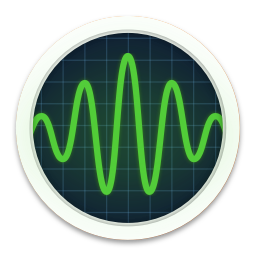BACK
 Lagrange Interpolation with time interval of day(s)       First date (optional)Produce file of the selected parametersDraw data   Dynamic graph   Show errors Spectral analysis (FFT, complex for 2D signal) Amplitude Power (amplitude2) min. frequency or period       max. frequency or period x linear scale x log scale y linear scale y log scale frequency spectrum (in cycle / unit of time) period spectrum (in unit of time) Weighted least square fit of periodic components (periods in unit of time) Positive periods         Polynomial of degree Negative periods       Weighted least square (take negative periods only for 2 dimensional signal)         Draw residuals and input data     Draw fit and input data     Print residuals In-phase and out-of-phase terms (a, b) are estimated, as well as amplitude A and phase φ : 1-D :$$\small X = A \cos[2\pi/T (t-t_0) + \phi] = a \cos[2\pi/T (t-t_0)] + b \cos[2\pi/T (t-t_0)]$$ 2-D : $$\small X +i Y = A e^{i [2\pi/T (t-t_0) + \phi]$$ with the reference epoch $$\small t_0$$ = 1/1/2000 0hUT that is : $$\small X = a \cos[2\pi/T (t-t_0)] - b \sin[2\pi /T (t-t_0)$$    $$\small Y = b cos[2\pi/T (t-t_0)] + a sin[2\pi/T (t-t_0) ]$$ with $$\small a = A \cos\phi$$      $$\small b = A\sin\phi$$ Vondrak low/high pass filter Remove parabolic trend Produce data file Draw   with input data Select band above Select band below (P0) time unit     Transfer coefficient for P0 :T0= % The Vondrak filter transfer function at another period P is given by : T=1/(1+(P0/P)6 (1-T0)/T0) For the case "Select band around" the periods in [P0 - 0.1*P0, P0 + 0.1*P0] are transmitted with the rate > T0%. For the case "Remove band around" the periods outside [P0 - 0.1*P0, P0 + 0.1*P0] are transmitted with the rate > T0 %. Panteleev band pass filter (for 2D signal) Produce data file Draw filtered data and enveloppe / phase referred to 2πfct   Select band around fc= cycle/time unit     Band width f0= cycle/time unit This band pass filter was designed by Russian astronomer and gravimetrist V. L. Panteleev. Its frequency transfer function is given by $$\small T(f) = \frac{f_0^4}{ ( f - f_c)^4 + f_0^4 }$$. At the edges of the window $$\small |f - f_c| = f_0$$ and $$\small T = 0.5$$. Singular Spectral Analysis (SSA) -  Zoom between and    The extracted components are decorellated over time windows of (in the time unit) with the interpolation lag (in the time unit). Firt step consists in the determination of the eigenvalues and eigenvectors printed by decreasing weight. Then 5 singular components are reconstructed according to the following combinations of eigenvectors, to be stated from the analysis of the eigenvalues . RC1 Reconstructed Component (RC) based upon eigenvectors N1 and N2 RC2 RC3 RC4 RC5    produce time series (date, signal, RC1,RC2,RC3,RC4,RC5,residuals) draw Graphic dimension x   output graphics png   pdf   ps       Partial Interface with the C-Fortran Libraries SLAVA (C. Bizouard) & MIMOSA (S. Lambert). Thank you for bringing to our knowledge any possible mistake, mail to : christian.bizouard at obspm.fr 1 Variations produced by the solid Earth zonal tides (IERS 2000 model) 2 TT=TAI+32.184 s (for parameter UT1-TAI) 3 Reference precession-nutation model for celestial pole offsets: either IAU 2000 or IAU 1980